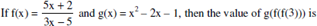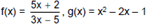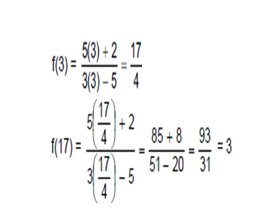# CAT 2017 – Slot 1 – Quantitative Ability – If the square of the 7th term of an arithmetic progression

Q. 1: If the square of the 7th term of an arithmetic progression with positive common difference equals the product of the 3rd and 17th terms, then the ratio of the first term to the common difference is
A) 2: 3
B) 3: 2
C) 3: 4
D) 4: 3

(a+6d)^2 = (a+2d)(a+16d)

a^2 + 12 ad + 36d^2 = a^2 + 18 ad + 32d^2

Since, d is positive,

We get the ratio of a:d = 2:3

Option (A)

##### Q. 2: In how many ways can 7 identical erasers be distributed among 4 kids in such a way that each kid gets at least one eraser but nobody gets more than 3 erasers?A) 16 B) 20 C) 14 D) 15

a + b + c + d = 7

Since, each kid gets 1 eraser, so a + b + c + d = 3

Now, no child can get more than 3 erasers.

There can be two cases. 2, 1, 0, 0 which can be represented in 4!/2! Ways = 12 ways

And 1,1,1,0 which can be represented in 4!/3! Ways = 4 ways

Option (A)

Q. 3:A) 2
B) 1/3
C) 6
D) 2/3

Explanation:-g(3) = 3^2 – 2(3) – 1 = 2.

Q. 4: Let a1, a2,……..a3n be an arithmetic progression with a1 = 3 and a2 = 7. If a1 + a2 + ….+a3n = 1830, then what is the smallest positive integer m such that m (a1 + a2 + …. + an ) > 1830?
A) 8
B) 9
C) 10
D) 11

a = 3

a + d = 7 => d=4

Applying formula of sum for AP

(3n/2) [6 + (3n-1)4] = 1830

On solving, we get n = 10

m>61/7

Max positive integer value of m = 9

Option (B)

##### Checkout Other Questions of CAT 2017 Slot 1 Paper:

Verbal Ability :              |   Q.01- Q.06  |  Q.07- Q.12  |  Q.13- Q.18  |  Q.19- Q.21  |  Q.22- Q.24  |  Q.25- Q.29  |  Q.30 – Q.34  |

Logical Reasoning :    |   Q.29 – Q.32  |

Quantitative Aptitude: |

### Free Material Area